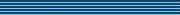### On the stabilization of discrete-time positive switched systems by means of Lyapunov based switching strategies

Speaker
Prof. M. E. Valcher
Duration
42:59
Date
19 February 2010
Abstract
Abstract: Positive switched systems typically arise to cope with two distinct modeling needs. On the one hand, switching among different models mathematically formalizes the fact that the system laws change under different operating conditions.On the other hand, the variables to be modeled may be quantities that have no meaning unless positive (temperatures, pressures,population levels, ...).

In this talk we consider the class of discrete-time positive switched systems, described, at each time t, by the first-order difference equation:

x(t+1) = A_{\sigma(t)} x(t),

where \sigma is a switching sequence, taking values in the finite set {1,2}, and for each index i, A_i is an n x n positive matrix. Assuming that both A_1 and A_2 are not Schur matrices, we focus on the stabilizability of the system, namely on the possibility of finding switching strategies that drive to zero the state evolution corresponding to every positive initial state x(0). To this end, we resort to state feedback switching laws, whose value at the time t depends on the value of some Lyapunov function in x(t).

We first explore quadratic positive definite functions, by extending a technique described by De Carlo et al.. Later, by taking advantage of the system positivity, we show that other classes of Lyapunov functions, such as linear copositive and quadratic copositive ones, may be used to design state-dependent stabilizing switching laws, and some of them may be designed under weaker conditions on the pair of matrices (A_1,A_2) with respect to those required for quadratic stabilizability.

Some comparisons between the performances of the switching strategies are given.
You should see the video here…
High quality
Fullscreen
Volume